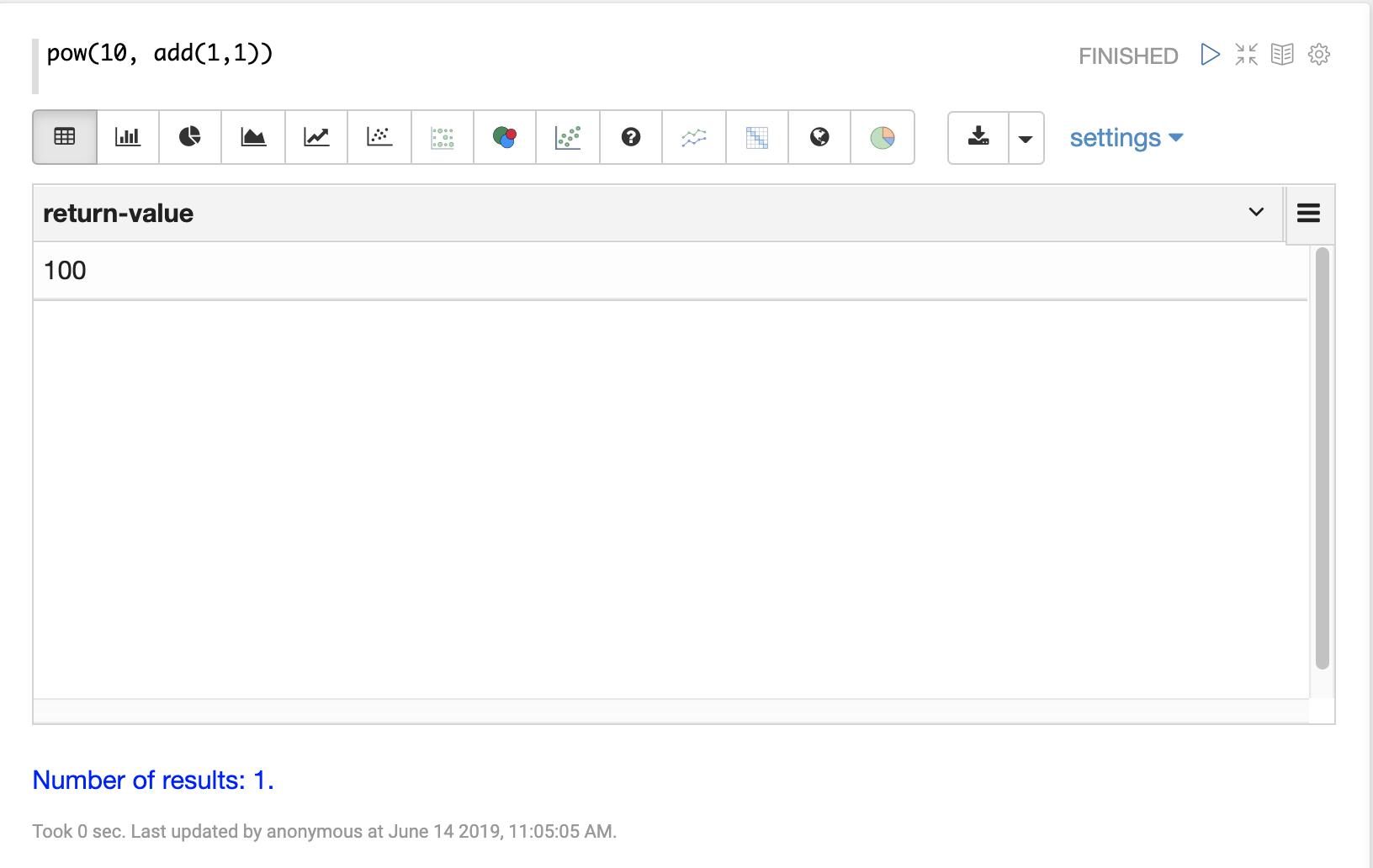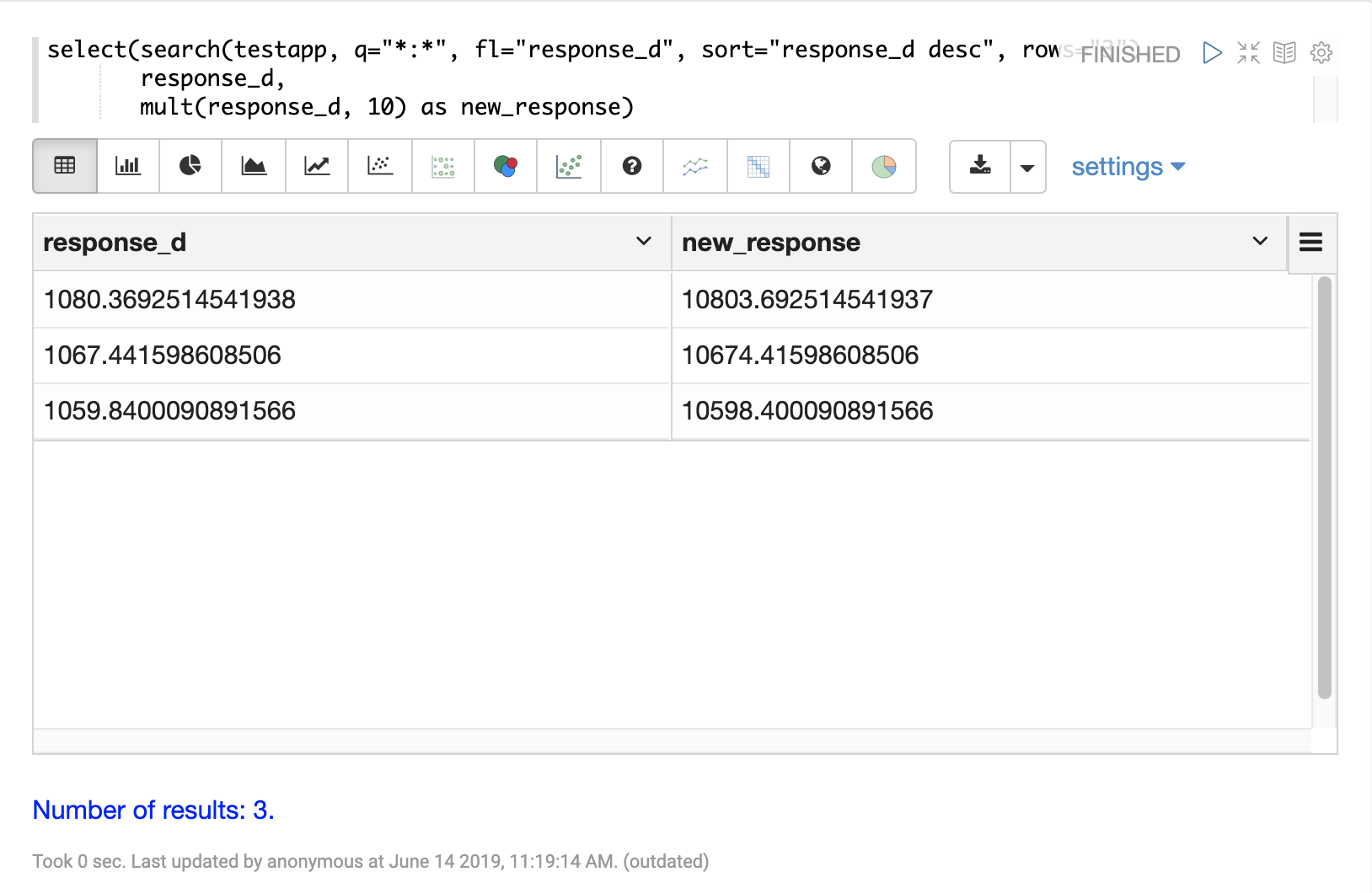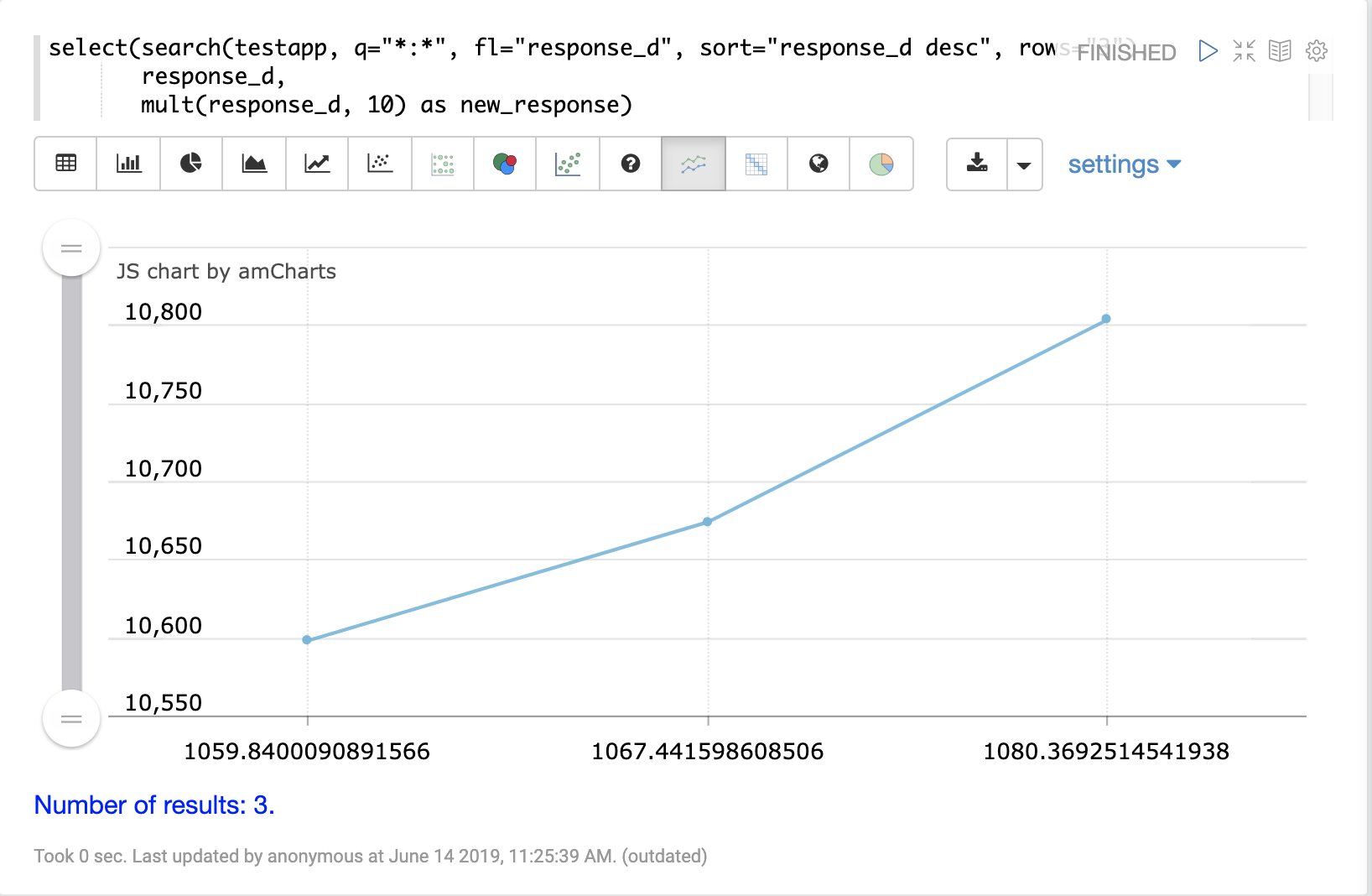# Scalar Math

The most basic math expressions are scalar expressions. Scalar expressions perform mathematical operations on numbers.

For example the expression below adds two numbers together:

``add(1, 1)``

When this expression is sent to the `/stream` handler it responds with:

``````{
"result-set": {
"docs": [
{
"return-value": 2
},
{
"EOF": true,
"RESPONSE_TIME": 2
}
]
}
}``````

Math expressions can be nested. For example in the expression below the output of the `add` function is the second parameter of the `pow` function:

``pow(10, add(1,1))``

This expression returns the following response:

``````{
"result-set": {
"docs": [
{
"return-value": 100
},
{
"EOF": true,
"RESPONSE_TIME": 0
}
]
}
}``````

## Visualization

In the Zeppelin-Solr interpreter you can simply type in scalar math functions and the result will be shown in a table format.The Number visualization can be used to visualize the number with text and icons.## Streaming Scalar Math

Scalar math expressions can also be applied to each tuple in a stream through use of the `select` stream decorator. The `select` function wraps a stream of tuples and selects fields to include in each tuple. The `select` function can also use math expressions to compute new values and add them to the outgoing tuples.

In the example below the `select` expression is wrapping a search expression. The `select` function is selecting the `response_d` field and computing a new field called `new_response` using the `mult` math expression.

The first parameter of the `mult` expression is the `response_d` field. The second parameter is the scalar value 10. This multiplies the value of the `response_d` field in each tuple by 10.

``````select(search(testapp, q="*:*", fl="response_d", sort="response_d desc", rows="3"),
response_d,
mult(response_d, 10) as new_response)``````

When this expression is sent to the `/stream` handler it responds with:

``````{
"result-set": {
"docs": [
{
"response_d": 1080.3692514541938,
"new_response": 10803.692514541937
},
{
"response_d": 1067.441598608506,
"new_response": 10674.41598608506
},
{
"response_d": 1059.8400090891566,
"new_response": 10598.400090891566
},
{
"EOF": true,
"RESPONSE_TIME": 12
}
]
}
}``````

## Visualization

The expression above can be visualized as a table using Zeppelin-Solr.By switching to one of the line chart visualizations the two variables can be plotted on the x and y-axis.## More Scalar Math Functions

The following scalar math functions are available in the math expressions library:

`abs`, `add`, `div`, `mult`, `sub`, `log`, `log10`, `pow`, `mod`, `ceil`, `floor`, `sin`, `asin`, `sinh`, `cos`, `acos`, `cosh`, `tan`, `atan`, `tanh`, `round`, `precision`, `recip`, `sqrt`, `cbrt`